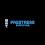# Electric Power class 10 | cbse24.com

## Electrical Power:

When an electric current flows through a conductor, electrical energy is used up and we say that the current is doing work.

Power is the rate at which energy is moved., so electrical power is the electrical work per unit time

### Unit of Power:

• So unit of power is Watt or another one is joule/second.
• Watt is a small unit KW, MW, GW is a bigger unit.
• 1KW=1000 Watt, 1MW = 1000000 Watt , 1GW=1000000000 Watt.• This electric bulb consumes electric energy at the rate of 100 joule/second in this picture , so its power is 100 watts.

### The formula for Calculating Electric Power:

• V=Potential difference(Voltage) in Volts
• I=Current in amperes
• The power expended in heating a resister or motor depends upon the potential difference between the terminals of the device and the electric current passing through it.
• The SI unit of power is the watt (W). One watt is the power consumed by the device rating 1A of current when operated at a potential difference of 1 V.

### Other formulae for calculating electric power:

#### (1) Power in terms of V and R.

P=V x I........................(1)

we know by ohm's law V/R=I put this in equation (1)

Note:-When V and R has given then electric power found by the formula

#### (2) Power P in terms of I and R

P=V x I........................(1)

we know by ohm's law V=I x R put this in equation (1)

Note:-When I and R has given then electric power found by the formula

### Power all formula:

1.2.3.